# A single neuron perceptron with sigmoid activation function – I – failure of gradient descent due to saturation

Readers who follow my series on a Python program for a "Multilayer Perceptron" [MLP] have noticed that I emphasized the importance of a normalization of input data in my last article:

A simple Python program for an ANN to cover the MNIST dataset – XII – accuracy evolution, learning rate, normalization

Our MLP "learned" by searching for the global minimum of the loss function via the "gradient descent" method. Normalization seemed to improve a smooth path to convergence whilst our algorithm moved into the direction of a global minimum on the surface of the loss or cost functions hyperplane over the weight parameter space. At least in our case where we used the sigmoid function as the activation function of the artificial neurons. I indicated that the reason for our observations had to do with properties of this function - especially for neurons in the first hidden layer of an MLP.

In case of the 4-layer MLP, which I used on the MNIST dataset, I applied a special form of normalization namely "standardization". I did this by using the StandarScaler of SciKit-Learn. See the following link for a description: Sklearn preprocessing StandardScaler

We saw the smoothing and helpful impact of normalization on the general convergence of gradient descent by the help of a few numerical experiments. The interaction of the normalization of 784 features with mini-batches and with a complicated 4 layer-MLP structure, which requires the determination of several hundreds of thousands weight values is however difficult to grasp or analyze. One understands that there is a basic relation to the properties of the activation function, but the sheer number of the dimensions of the feature and weight spaces and statistics make a thorough understanding difficult.

Since then I have thought a bit about how to set up a really small and comprehensible experiment which makes the positive impact of normalization visible in a direct and visual form. I have come up with the following most simple scenario, namely the training of a simple perceptron with only one computing neuron and two additional "stupid" neurons in an input layer which just feed input data to our computing neuron: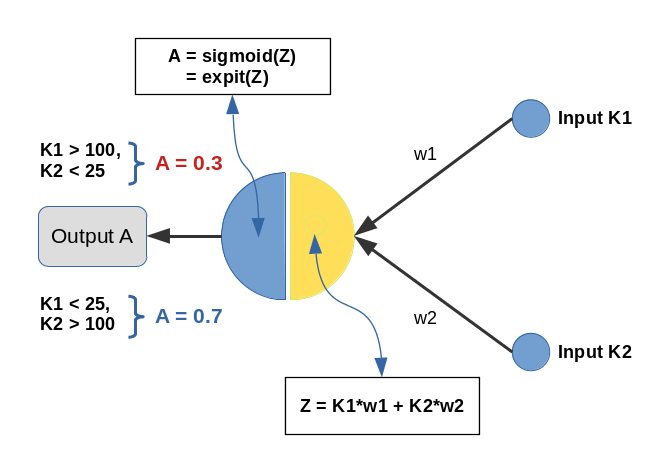Input values K1 and K2 are multiplied by weights w1 and w2 and added by the central solitary "neuron". We use the sigmoid function as the "activation function" of this neuron - well anticipating that the properties of this function may lead to trouble.

The perceptron has only one task: Discriminate between two different types of input data by assigning them two distinct output values.

• For K1 > 100 and K2 < 25 we want an output of A=0.3.
• For K1 &l; 25 and K2 > 100 we, instead, want an output of A=0.7

We shall feed the perceptron only 14 different pairs of input values K1[i], K2[i] (i =0,1,..13), e.g. in form of lists:

```li_K1 = [200.0,   1.0, 160.0,  11.0, 220.0,  11.0, 120.0,  22.0, 195.0,  15.0, 130.0,   5.0, 185.0,  16.0]
li_K2 = [ 14.0, 107.0,  10.0, 193.0,  32.0, 178.0,   2.0, 210.0,  12.0, 134.0,  15.0, 167.0,  10.0, 229.0]
```

(The careful reader detects one dirty example li_K2 = 32 (> 25), in contrast to our setting. Just to see how much of an impact such a deviation has ...)

We call each pair (K1, K2)=(li_K1[i], li_K2[i]) for a give "i" a "sample". Each sample contains values for two "features": K1 and K2. So, our solitary computing neuron has to solve a typical classification problem - it shall distinguish between two groups of input samples. In a way it must learn the difference between small and big numbers for 2 situations appearing at its input channels.

Off topic: This morning I listened to a series of comments of Mr. Trump during the last weeks on the development of the Corona virus crisis in the USA. Afterwards, I decided to dedicate this mini-series of articles on a perceptron to him - a person who claims to "maybe" be "natural talent" on complicated things as epidemic developments. Enjoy (?) his own words via an audio compilation in the following news article:
https://www.faz.net/aktuell/politik/trumps-praesidentschaft/usa-zehn-wochen-corona-in-den-worten-von-trump-16708603.html

# Two well separable clusters

In the 2-dim feature space {K1, K2} we have just two clusters of input data: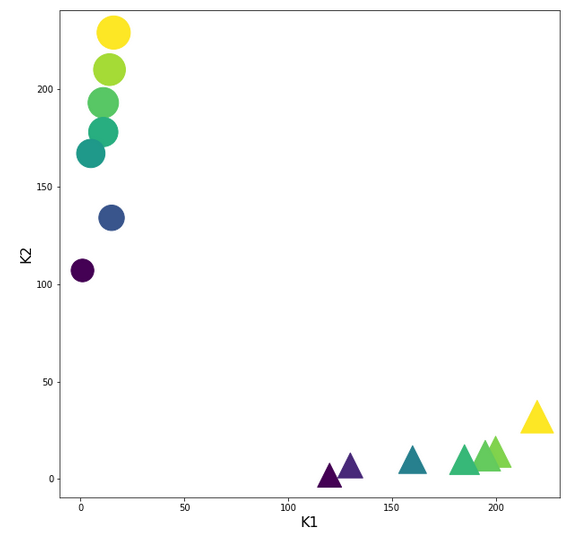Each cluster has a long diameter along one of the feature axes - but overall the clusters are well separable. A rather good separation surface would e.g. be a diagonal line.

For a given input sample with K-values K1 and K2 we define the output of our computing neuron to be

A(w1, w2) = expit( w1*K1 + w2*K2 ) ,

where expit() symbolizes the sigmoid function. See the next section for more details.

Corresponding target-values for the output A are (with at1 = 0.3 and at2 = 0.7):

```li_a_tgt = [at1,  at2,  at1,  at2,  at1,  at2,  at1,  at2,  at1,  at2,  at1,  at2,   at1,  at2]
```

With the help of these target values our poor neuron shall learn from the 14 input samples to which cluster a given future sample probably belongs to. We shall use the "gradient descent" method to train the neuron for this classification task. o solve the task our neuron must find a reasonable separation surface - a line - in the {K1,K2}-plane; but it got the additional task to associate two distinct output values "A" with the two clusters:

A=0.7 for data points with a large K1-value and A=0.3 for data points with a small K1-value.

So, the separation surface has to fulfill some side conditions.

Readers with a background in Machine Learning and MLPs will now ask: Why did you pick the special values 0.3 and 0.7? A good question - I will come back to it during our experiments. Another even more critical question could be: What about a bias neuron in the input layer? Don't we need it? Again, a very good question! A bias neuron allows for a shift of a separation surface in the feature space. But due to the almost symmetrical nature of our input data (see the positions and orientations of the clusters!) and the target values the impact of a bias neuron on the end result would probably only be small - but we shall come back to the topic of a bias neuron in a later article. But you are free to extend the codes given below to account for a bias neuron in the input layer. You will notice a significant impact if you change either the relative symmetry of the input or of the output data. But lets keep things simple for the next hours ...

# The sigmoid function and its saturation for big arguments

You can import the sigmoid function under the name "expit" from the "scipy" library into your Python code. The sigmoid function is a smooth one - but it quickly saturates for big negative or positive values: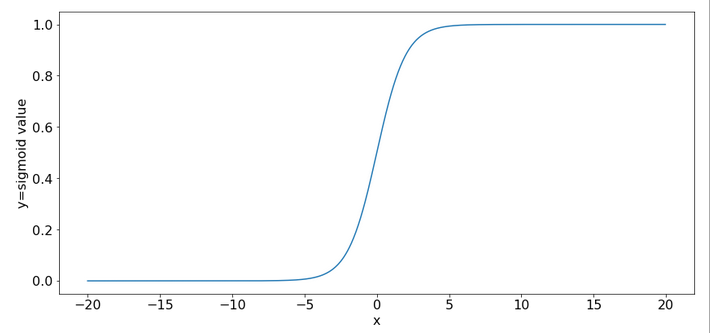So, output values get almost indistinguishable if the absolute values of the arguments are bigger than 15.

# What is interesting about our input data? What is the relation to MNIST data?

The special situation about the features in our example is the following: For one and the same feature we have a big number and a small number to work with - depending on the sample. Which feature value - K1 or K2 - is big depends on the sample.

This is something that also happens with the input "features" (i.e. pixels) coming from a MNIST-image:
For a certain feature (= a pixel at a defined position in the 28x28 picture) in a given sample (= a distinct image) we may get a big number as 255. For another feature (= pixel) the situation may be totally different and we may find a value of 0. In another sample (= picture) we may get the reverse situation for the same two pixels.

What happens in such a situation at a specific neuron in the first hidden neuron layer of a MLP when we start gradient descent with a statistical distribution of weight values? If we are unlucky then the initial statistical weight constellation for a sample may lead to a big number of the total input to our selected hidden neuron with the effect of a very small gradient at this node - due to saturation of the sigmoid function.

To give you a feeling: Assume that you have statistical weights in the range of [-0.025, 0.025]. Assume further that only 4 pixels of a MNIST picture with a value of 200 contribute with a local maximum weight of 0.25; then we get a a minimum input at our node of 4*0.25*200 = 20. The gradient of expit(20) has a value of 2e-9. Even if we multiply by the required factor of 200 for a weight correction at one of the contributing input nodes we would would arrive at 4e-7. Quite hopeless. Of course, the situation is not that bad for all weights and image-samples, but you get an idea about the basic problem ....

Our simple scenario breaks the MNIST situation down to just two features and just one working neuron - and therefore makes the correction situation for gradient descent really extreme - but interesting, too. And we can analyze the situation far better than for a MLP because we deal with an only 2-dimensional feature-space {K1, K2} and a 2-dimensional weight-space {w1, w2}.

# A simple quadratic cost function for our neuron

For given values w1 and w2, i.e. a tuple (w1, w2), we define a quadratic cost or loss function C_sgl for one single input sample (K1, K2) as follows:

C_sgl = 0.5 * ( li_a_tgt(i) - expit(z_i) )**2, with z_i = li_K[i]*w1 + li_K2[i]*w2

The total cost-function for the batch of all 14 samples is just the sum of all these terms for the individual samples.

# Existence of a solution for our posed problem?

From all we theoretically know about the properties of a simple perceptron it should be able to find a reasonable solution! But, how do we know that a reasonable solution for a (w1, w2)-pair does exist at all? One line of reasoning goes like follows:

For two samples - each a member of either cluster - you can plot the hyperplanes of the related outputs "A(K1, K2) = expit(w1*K1+w2*K2)" over the (w1, w2)-space. These hyperplanes are almost orthogonal to each other. If you project the curves of a cut with the A=0.3-planes and the A=0.7-planes down to the (w1, w2)-plane at the center you get straight lines - almost orthogonally oriented against each other. So, such 2 specific lines cut each other in a well defined point - somewhere off the center. As the expit()-function is a relatively steep one for our big input values the crossing point is relatively close to the center. If we choose other samples we would get slightly different lines and different crossing points of the projected lines - but not too far from each other.

The next plot shows the expit()-functions close to the center of the (w1, w2)-plane for two specific samples of either cluster. We have in addition displayed the surfaces for A=0.7 and A=0.3.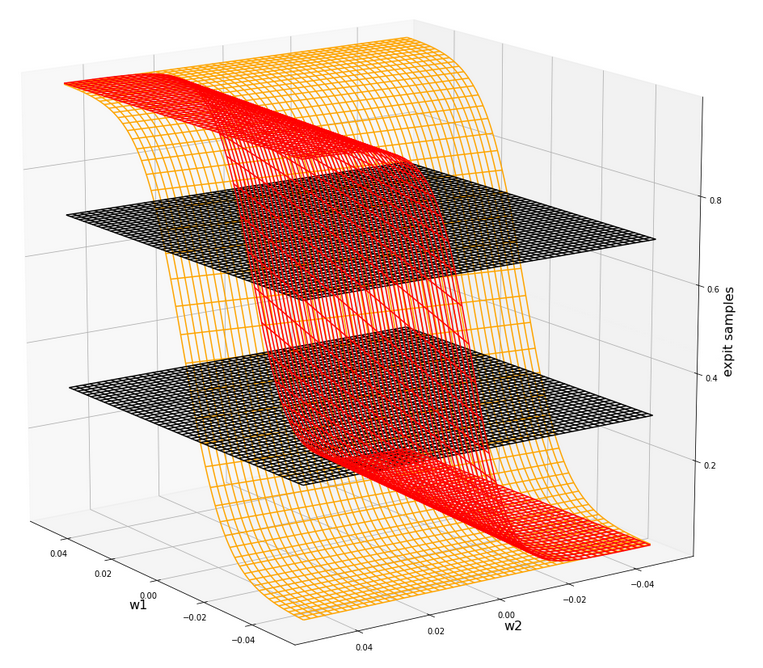The following plot shows the projections of the cuts of the surfaces for 7 samples of each cluster with the A=0.3-plane and the A=0.7, respectively.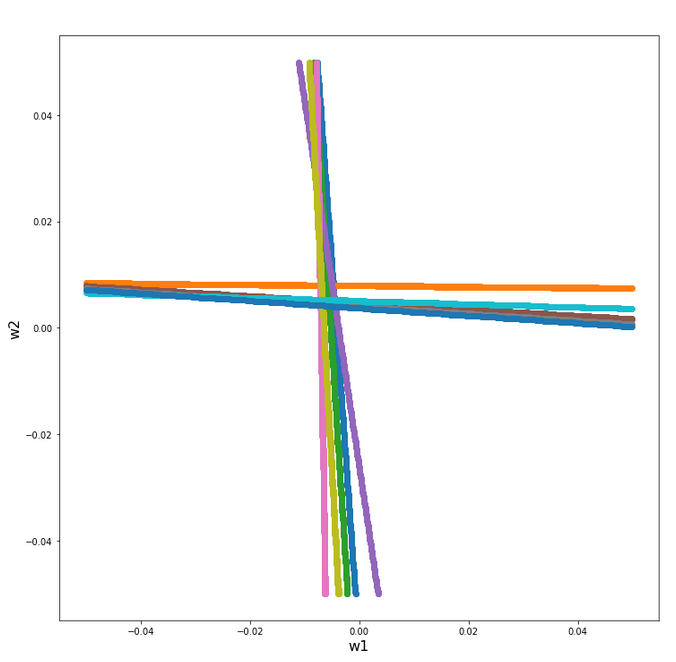The area of crossings is not too big on the chosen scale of w1, w2. Looking at the graphics we would expect an optimal point around (w1=-0.005, w2=+0.005) - for the original, unnormalized input data.

By the way: There is also a solution for at1=0.3 and at2=0.3, but a strange one. Such a setup would not allow for discrimination. We expect a rather strange behavior then. A first guess could be: The resulting separation curve in the (K1, K2)-plane would move out of the area between the two clusters.

# Code for a mesh based display of the costs over the weight-parameter space

Below you find the code suited for a Jupyter cell to get a mesh display of the cost values

```import numpy as np
import numpy as np
import random
import math
import sys

from sklearn.preprocessing import StandardScaler
from sklearn.preprocessing import Normalizer
from sklearn.preprocessing import MinMaxScaler
from scipy.special import expit

import matplotlib as mpl
from matplotlib import pyplot as plt
from matplotlib.colors import ListedColormap
import matplotlib.patches as mpat
from mpl_toolkits import mplot3d
from mpl_toolkits.mplot3d import Axes3D

# total cost functions for overview
# *********************************
def costs_mesh(num_samples, W1, W2, li_K1, li_K2, li_a_tgt):
zshape = W1.shape
li_Z_sgl = []
li_C_sgl = []
C = np.zeros(zshape)

rg_idx = range(num_samples)
for idx in rg_idx:
Z_idx      = W1 * li_K1[idx] + W2 * li_K2[idx]
A_tgt_idx  = li_a_tgt[idx] * np.ones(zshape)
C_idx = 0.5 * ( A_tgt_idx - expit(Z_idx) )**2
li_C_sgl.append( C_idx )
C += C_idx

C /= np.float(num_samples)
return C, li_C_sgl

# ******************
# Simple Perceptron
#*******************

# input at 2 nodes => 2 features K1 and K2 => there will be just one output neuron
li_K1 = [200.0,   1.0, 160.0,  11.0, 220.0,  11.0, 120.0,  14.0, 195.0,  15.0, 130.0,   5.0, 185.0,  16.0  ]
li_K2 = [ 14.0, 107.0,  10.0, 193.0,  32.0, 178.0,   2.0, 210.0,  12.0, 134.0,  7.0, 167.0,  10.0, 229.0 ]

# target values
at1 = 0.3; at2 = 0.7
li_a_tgt  = [at1,  at2,  at1,  at2,   at1,   at2,  at1,   at2,  at1,  at2,  at1,  at2,   at1,  at2 ]

# Change to np floats
li_K1 = np.array(li_K1)
li_K2 = np.array(li_K2)
li_a_tgt = np.array(li_a_tgt)

num_samples = len(li_K1)

# Get overview over costs on mesh
# *******************************
# Mesh of weight values
wm1 = np.arange(-0.2,0.4,0.002)
wm2 = np.arange(-0.2,0.2,0.002)
W1, W2 = np.meshgrid(wm1, wm2)
# costs
C, li_C_sgl  = costs_mesh(num_samples=num_samples, W1=W1, W2=W2, \
li_K1=li_K1, li_K2=li_K2, li_a_tgt = li_a_tgt)

# Mesh-Plots
# ********
fig_size = plt.rcParams["figure.figsize"]
print(fig_size)
fig_size = 19
fig_size = 19

fig1 = plt.figure(1)
fig2 = plt.figure(2)

ax1 = fig1.gca(projection='3d')
ax1.get_proj = lambda: np.dot(Axes3D.get_proj(ax1), np.diag([1.0, 1.0, 1, 1]))
ax1.view_init(15,148)
ax1.set_xlabel('w1', fontsize=16)
ax1.set_ylabel('w2', fontsize=16)
ax1.set_zlabel('single costs', fontsize=16)

#ax1.plot_wireframe(W1, W2, li_C_sgl, colors=('blue'))
#ax1.plot_wireframe(W1, W2, li_C_sgl, colors=('orange'))
ax1.plot_wireframe(W1, W2, li_C_sgl, colors=('orange'))
ax1.plot_wireframe(W1, W2, li_C_sgl, colors=('green'))
#ax1.plot_wireframe(W1, W2, li_C_sgl, colors=('orange'))
#ax1.plot_wireframe(W1, W2, li_C_sgl, colors=('magenta'))

ax2 = fig2.gca(projection='3d')
ax2.get_proj = lambda: np.dot(Axes3D.get_proj(ax2), np.diag([1.0, 1.0, 1, 1]))
ax2.view_init(15,140)
ax2.set_xlabel('w1', fontsize=16)
ax2.set_ylabel('w2', fontsize=16)
ax2.set_zlabel('Total costs', fontsize=16)
ax2.plot_wireframe(W1, W2, 1.2*C, colors=('green'))

```

# The cost landscape for individual samples without normalization

Gradient descent tries to find a minimum of a cost function by varying the weight values systematically in the cost gradient's direction. To get an overview about the cost hyperplane over the 2-dim (w1, w2)-space we make some plots. Let us first plot 2 the individual costs for the input samples i=0 and i=5.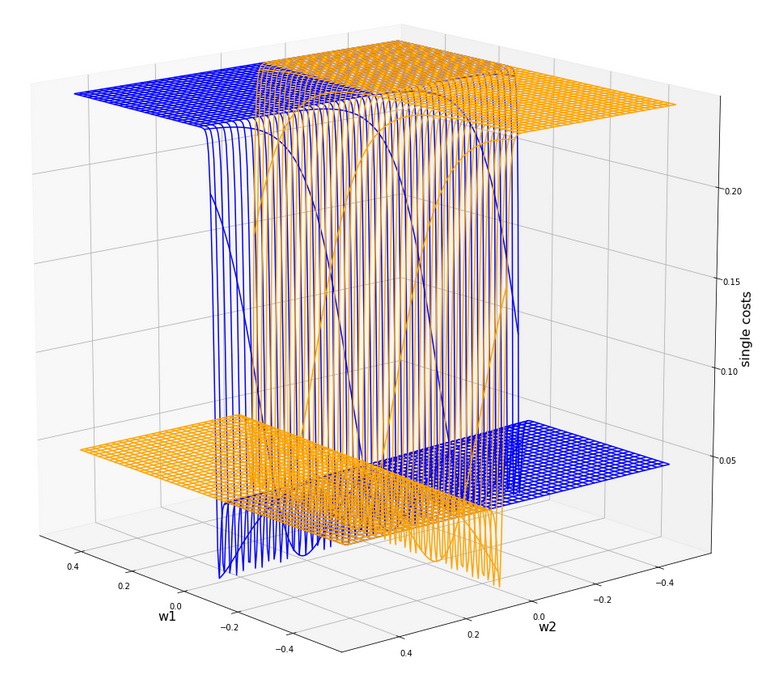Actually the cost functions for the different samples do show some systematic, though small differences. Try it out yourself ... Here is the plot for samples 1,5,9 (counted from 0!).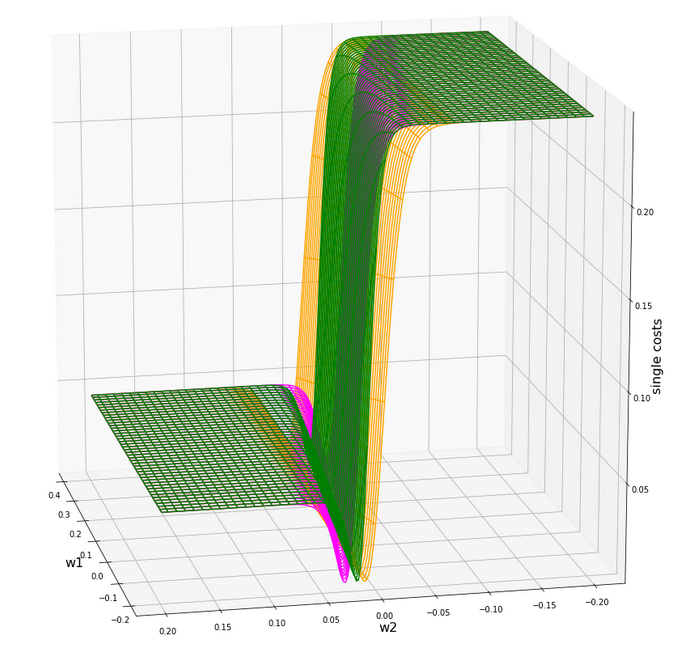You see different orientation angles in the (w1, w2)-plane?

# Total cost landscape without normalization

Now let us look at the total costs; to arrive at a comparable level with the other plots I divided the sum by 14: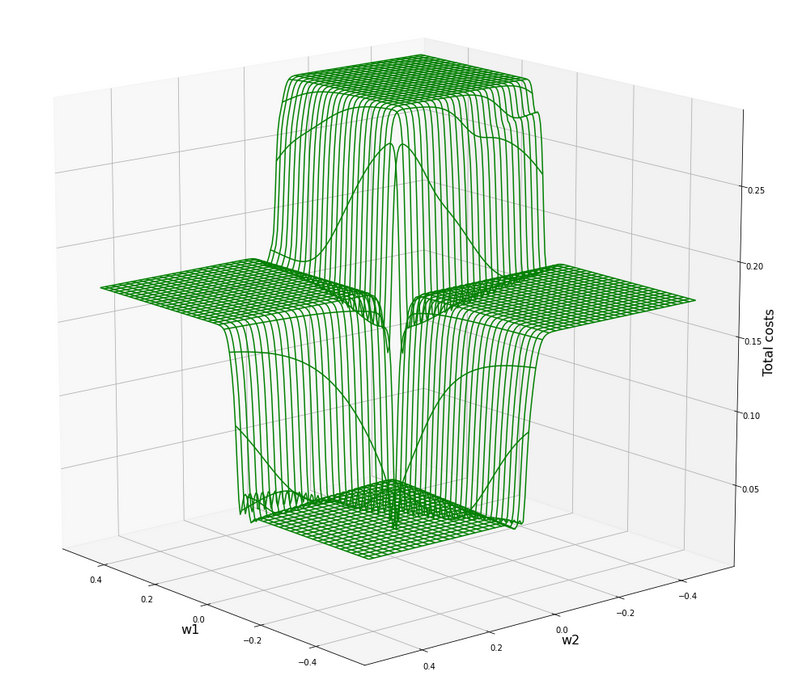All of the above cost plots look like big trouble for both the "stochastic gradient descent" and the "batch gradient descent" methods for "Machine Learning" [ML]:

We clearly see the effect of the sigmoid saturation. We get almost flat areas beyond certain relatively small w1- and w2-values (|w1| > 0.02, |w2| > 0.02). The gradients in this areas will be very, very close to zero. So, if we have a starting point as e.g. (w1=0.3, w2=0.2) our gradient descent would get stuck. Due to the big input values of at least one feature.

In the center of the {w1, w2}-plane, however, we detect a steep slope to a global minimum.

But how to get there? Let us say, we start with w1=0.04, w2=0.04. The learning-rate "η" is used to correct the weight values by

In the beginning you would need a big "η" to get closer to the center due to small gradient values. However, if you choose it too big you may pass the tiny area of the minimum and just hop to an area of a different cost level with again a gradient close to zero. But you cannot decrease the learning rate fast as a remedy, either, to avoid getting stuck again.

# A view at the center of the loss function hyperplane

Let us take a closer look at the center of our disastrous total cost function. We can get there by reducing our mesh to a region defined by "-0.05 < w1, w2 < 0.05". We get :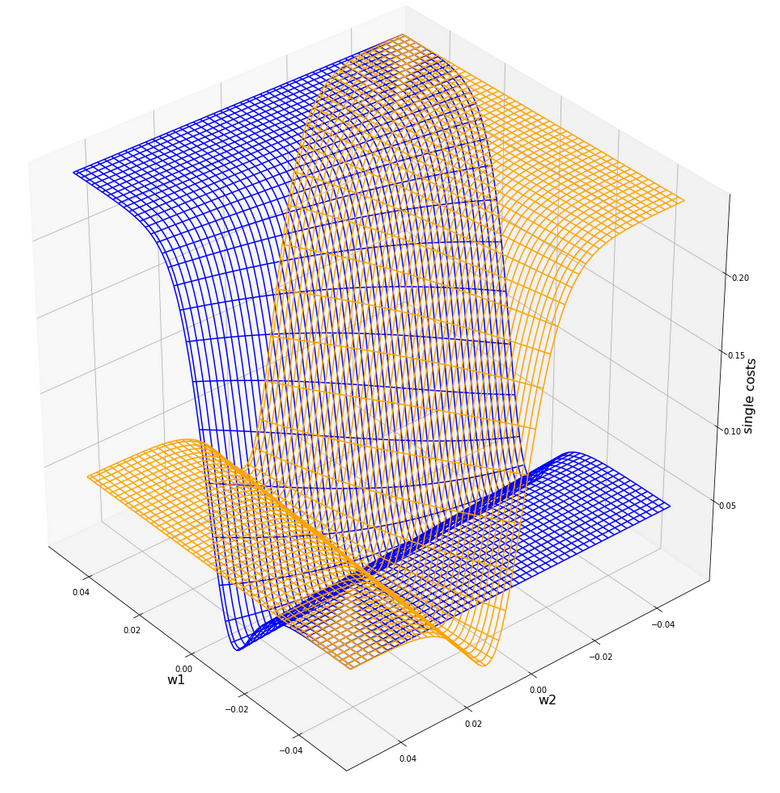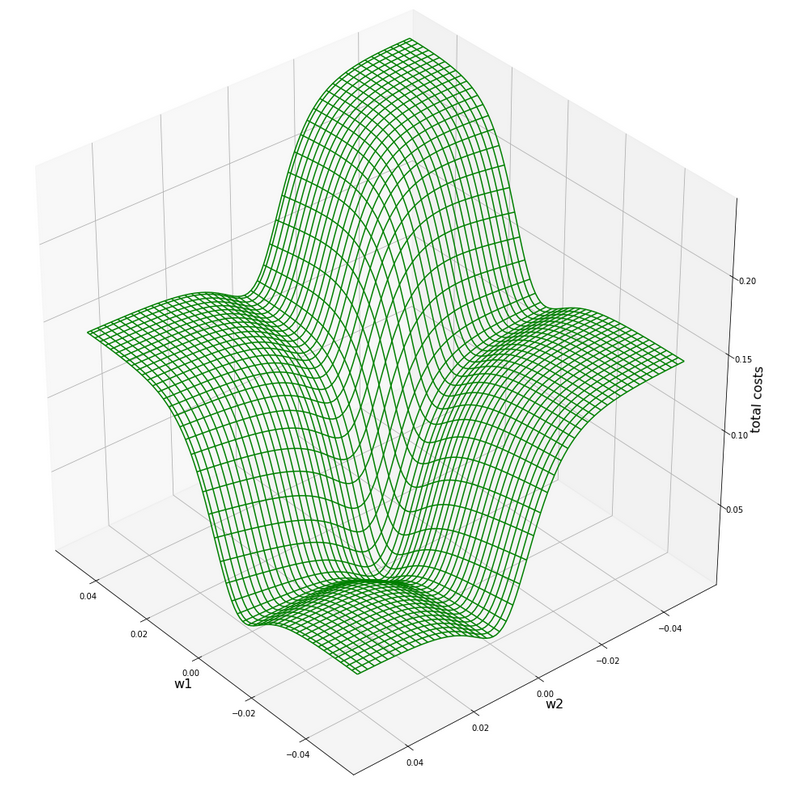This looks actually much better - on such a surface we could probably work with gradient descent. There is a clear minimum visible - and on this scale of the (w1, w2)-values we also recognize reasonably paths into this minimum. An analysis of the meshdata to get values for the minimum is possible by the following statements:

```print("min =", C.min())
pt_min = np.argwhere(C==C.min())
w1=W1[pt_min][pt_min]
w2=W2[pt_min][pt_min]
print("w1 = ", w1)
print("w2 = ", w2)
```

The result is:

min = 0.0006446277000906343
w1 = -0.004999999999999963
w2 = 0.005000000000000046

But to approach this minimum by a smooth gradient descent we would have had to know in advance at what tiny values of (w1, w2) to start with gradient descent - and choose our η suitably small in addition. This is easy in our most simplistic one neuron case, but you almost never can fulfill the first condition when dealing with real artificial neural networks for complex scenarios.

And a naive gradient descent with a standard choice of a (w1, w2)-starting point would have lead us to nowhere in our one-neuron case - as we shall see in a minute ..

Let us keep one question in mind for a while: Is there a chance that we could get the hyperplane surface to look similar to the one at the center - but for much bigger weight values?

# Some Python code for gradient descent for our one neuron scenario

Here are some useful functions, which we shall use later on to perform a gradient descent:

```
# ****************************************
# Functions for stochastic GRADIENT DESCENT
# *****************************************
import random
import pandas as pd

# derivative of expit
def d_expit(z):
exz = expit(z)
dex = exz * (1.0 - exz)
return dex

# single costs for stochastic descent
# ************************************
def dcost_sgl(w1, w2, idx, li_K1, li_K2, li_a_tgt):
z_in  = w1 * li_K1[idx] + w2 * li_K2[idx]
a_tgt = li_a_tgt[idx]
c = 0.5 * ( a_tgt - expit(z_in))**2
return c

# *********
def grad_sgl(w1, w2, idx, li_K1, li_K2, li_a_tgt):
z_in  = w1 * li_K1[idx] + w2 * li_K2[idx]
a_tgt = li_a_tgt[idx]
gradw1 = 0.5 * 2.0 * (a_tgt - expit(z_in)) * (-d_expit(z_in)) * li_K1[idx]
gradw2 = 0.5 * 2.0 * (a_tgt - expit(z_in)) * (-d_expit(z_in)) * li_K2[idx]

def grad_tot(num_samples, w1, w2, li_K1, li_K2, li_a_tgt):
rg_idx = range(num_samples)
for idx in rg_idx:
z_in  = w1 * li_K1[idx] + w2 * li_K2[idx]
a_tgt = li_a_tgt[idx]
gradw1_idx = 0.5 * 2.0 * (a_tgt - expit(z_in)) * (-d_expit(z_in)) * li_K1[idx]
gradw2_idx = 0.5 * 2.0 * (a_tgt - expit(z_in)) * (-d_expit(z_in)) * li_K2[idx]

# total costs at given point
# ************************************
def dcost_tot(num_samples, w1, w2,li_K1, li_K2, li_a_tgt):
c_tot  = 0
rg_idx = range(num_samples)
for idx in rg_idx:
#z_in  = w1 * li_K1[idx] + w2 * li_K2[idx]
a_tgt = li_a_tgt[idx]
c_idx = dcost_sgl(w1, w2, idx, li_K1, li_K2, li_a_tgt)
c_tot += c_idx
ctot = 1.0/num_samples * c_tot
return c_tot

# Prediction function
# ********************
def predict_batch(num_samples, w1, w2,ay_k_1, ay_k_2, li_a_tgt):
shape_res = (num_samples, 5)
ResData = np.zeros(shape_res)
rg_idx = range(num_samples)
err = 0.0
for idx in rg_idx:
z_in  = w1 * ay_k_1[idx] + w2 * ay_k_2[idx]
a_out = expit(z_in)
a_tgt = li_a_tgt[idx]
err_idx = np.absolute(a_out - a_tgt) / a_tgt
err += err_idx
ResData[idx] = ay_k_1[idx]
ResData[idx] = ay_k_2[idx]
ResData[idx] = a_tgt
ResData[idx] = a_out
ResData[idx] = err_idx
err /= float(num_samples)
return err, ResData

def predict_sgl(k1, k2, w1, w2):
z_in  = w1 * k1 + w2 * k2
a_out = expit(z_in)
return a_out

def create_df(ResData):
''' ResData: Array with result values K1, K2, Tgt, A, rel.err
'''
cols=["K1", "K2", "Tgt", "Res", "Err"]
df = pd.DataFrame(ResData, columns=cols)
return df

```

With these functions a quick and dirty "gradient descent" can be achieved by the following code:

```
# **********************************
# Quick and dirty Gradient Descent
# **********************************
b_scale_2 = False
if b_scale_2:
ay_k_1 = ay_K1
ay_k_2 = ay_K2
else:
ay_k_1 = li_K1
ay_k_2 = li_K2

li_w1_st = []
li_w2_st = []
li_c_sgl_st = []
li_c_tot_st = []

li_w1_ba = []
li_w2_ba = []
li_c_sgl_ba = []
li_c_tot_ba = []

idxc = 2

# Starting point
#***************
w1_start = -0.04
w2_start = -0.0455
#w1_start = 0.5
#w2_start = -0.5

# learn rate
# **********
eta = 0.0001
decrease_rate = 0.000000001
num_steps = 2500

# norm = 1
#eta = 0.75
#decrease_rate = 0.000000001
#num_steps = 100

# *********************
rg_j = range(num_steps)
rg_i = range(num_samples)
w1d_st = w1_start
w2d_st = w2_start
w1d_ba = w1_start
w2d_ba = w2_start

for j in rg_j:
eta = eta / (1.0 + float(j) * decrease_rate)
# loop over samples and individ. corrs
ns = num_samples
rg = range(ns)
rg_idx = random.sample(rg, num_samples)
#print("\n")
for idx in rg_idx:
#print("idx = ", idx)
li_w1_st.append(w1d_st)
li_w2_st.append(w2d_st)

# costs for special sample
cd_sgl_st = dcost_sgl(w1d_st, w2d_st, idx, ay_k_1, ay_k_2, li_a_tgt)
li_c_sgl_st.append(cd_sgl_st)

# total costs for special sample
cd_tot_st = dcost_tot(num_samples, w1d_st, w2d_st, ay_k_1, ay_k_2, li_a_tgt)
li_c_tot_st.append(cd_tot_st)
#print("j:", j, " li_c_tot[j] = ", li_c_tot[j] )

# work with total costs and total gradient
li_w1_ba.append(w1d_ba)
li_w2_ba.append(w2d_ba)
co_ba = dcost_tot(num_samples, w1d_ba, w2d_ba, ay_k_1, ay_k_2, li_a_tgt)
li_c_tot_ba.append(co_ba)

# Printed Output
# ***************
num_end = len(li_w1_st)
err_sgl, ResData_sgl = predict_batch(num_samples, li_w1_st[num_end-1], li_w2_st[num_end-1], ay_k_1, ay_k_2, li_a_tgt)
err_ba,  ResData_ba = predict_batch(num_samples, li_w1_ba[num_steps-1], li_w2_ba[num_steps-1], ay_k_1, ay_k_2, li_a_tgt)
df_sgl = create_df(ResData_sgl)
df_ba  = create_df(ResData_ba)
print("\n", df_sgl)
print("\n", df_ba)
print("\nTotal error stoch descent: ", err_sgl )
print("Total error batch descent: ", err_ba )

# Styled Pandas Output
# *******************
df_ba

```

Those readers who followed my series on a Multilayer Perceptron should have no difficulties to understand the code: I used two methods in parallel - one for a "stochastic descent" and one for a "batch descent":

• During "stochastic descent" we correct the weights by a stepwise application of the cost-gradients of single samples. (We shuffle the order of the samples statistically during epochs to avoid cyclic effects.) This is done for all samples during an epoch.
• During batch gradient we apply the gradient of the total costs of all samples once during each epoch.

And here is also some code to perform some plotting after training runs:

```
# Plots for Single neuron Gradient Descent
# ****************************************
#sizing
fig_size = plt.rcParams["figure.figsize"]
fig_size = 14
fig_size = 5

fig1 = plt.figure(1)
fig2 = plt.figure(2)
fig3 = plt.figure(3)
fig4 = plt.figure(4)

ax1_1.plot(range(len(li_c_sgl_st)), li_c_sgl_st)
#ax1_1.set_xlim (0, num_tot+5)
#ax1_1.set_ylim (0, 0.4)
ax1_1.set_xlabel("epochs * batches (" + str(num_steps) + " * " + str(num_samples) + " )")
ax1_1.set_ylabel("sgl costs")

ax1_2.plot(range(len(li_c_tot_st)), li_c_tot_st)
#ax1_2.set_xlim (0, num_tot+5)
#ax1_2.set_ylim (0, y_max_err)
ax1_2.set_xlabel("epochs * batches (" + str(num_steps) + " * " + str(num_samples) + " )")
ax1_2.set_ylabel("total costs ")

ax2_1.plot(range(len(li_w1_st)), li_w1_st)
#ax1_1.set_xlim (0, num_tot+5)
#ax1_1.set_ylim (0, y_max_costs)
ax2_1.set_xlabel("epochs * batches (" + str(num_steps) + " * " + str(num_samples) + " )")
ax2_1.set_ylabel("w1")

ax2_2.plot(range(len(li_w2_st)), li_w2_st)
#ax1_2.set_xlim (0, num_to_t+5)
#ax1_2.set_ylim (0, y_max_err)
ax2_2.set_xlabel("epochs * batches (" + str(num_steps) + " * " + str(num_samples) + " )")
ax2_2.set_ylabel("w2")

ax3_1.plot(range(len(li_c_tot_ba)), li_c_tot_ba)
#ax3_1.set_xlim (0, num_tot+5)
#ax3_1.set_ylim (0, 0.4)
ax3_1.set_xlabel("epochs (" + str(num_steps) + " )")
ax3_1.set_ylabel("batch costs")

ax4_1.plot(range(len(li_w1_ba)), li_w1_ba)
#ax4_1.set_xlim (0, num_tot+5)
#ax4_1.set_ylim (0, y_max_costs)
ax4_1.set_xlabel("epochs (" + str(num_steps) + " )")
ax4_1.set_ylabel("w1")

ax4_2.plot(range(len(li_w2_ba)), li_w2_ba)
#ax4_2.set_xlim (0, num_to_t+5)
#ax4_2.set_ylim (0, y_max_err)
ax4_2.set_xlabel("epochs (" + str(num_steps) + " )")
ax4_2.set_ylabel("w2")

```

You can put these codes into suitable cells of a Jupyter environment and start doing experiments on your PC.

# Frustration WITHOUT data normalization ...

Let us get the worst behind us:
Let us use un-normalized input data, set a standard starting point for the weights and try a gradient descent with 2500 epochs.
Well, what are standard initial weight values? We can follow LeCun's advice on bigger networks: a uniform distribution between - sqrt(1/2) and +srt(1/2) = 0.7 should be helpful. Well, we take such values. The parameters of our trial run are:

w1_start = -0.1, w2_start = 0.1, eta = 0.01, decrease_rate = 0.000000001, num_steps = 12500

You, unfortunately, get nowhere:

```        K1     K2  Tgt           Res       Err
0   200.0   14.0  0.3  3.124346e-15  1.000000
1     1.0  107.0  0.7  9.999996e-01  0.428571
2   160.0   10.0  0.3  2.104822e-12  1.000000
3    11.0  193.0  0.7  1.000000e+00  0.428571
4   220.0   32.0  0.3  1.117954e-15  1.000000
5    11.0  178.0  0.7  1.000000e+00  0.428571
6   120.0    2.0  0.3  8.122661e-10  1.000000
7    14.0  210.0  0.7  1.000000e+00  0.428571
8   195.0   12.0  0.3  5.722374e-15  1.000000
9    15.0  134.0  0.7  9.999999e-01  0.428571
10  130.0    7.0  0.3  2.783284e-10  1.000000
11    5.0  167.0  0.7  1.000000e+00  0.428571
12  185.0   10.0  0.3  2.536279e-14  1.000000
13   16.0  229.0  0.7  1.000000e+00  0.428571

K1     K2  Tgt           Res       Err
0   200.0   14.0  0.3  7.567897e-24  1.000000
1     1.0  107.0  0.7  1.000000e+00  0.428571
2   160.0   10.0  0.3  1.485593e-19  1.000000
3    11.0  193.0  0.7  1.000000e+00  0.428571
4   220.0   32.0  0.3  1.411189e-21  1.000000
5    11.0  178.0  0.7  1.000000e+00  0.428571
6   120.0    2.0  0.3  2.293804e-16  1.000000
7    14.0  210.0  0.7  1.000000e+00  0.428571
8   195.0   12.0  0.3  1.003437e-23  1.000000
9    15.0  134.0  0.7  1.000000e+00  0.428571
10  130.0    7.0  0.3  2.463730e-16  1.000000
11    5.0  167.0  0.7  1.000000e+00  0.428571
12  185.0   10.0  0.3  6.290055e-23  1.000000
13   16.0  229.0  0.7  1.000000e+00  0.428571

Total error stoch descent:  0.7142856616691734
Total error batch descent:  0.7142857142857143
```

A parameter setting like

w1_start = -0.1, w2_start = 0.1, eta = 0.0001, decrease_rate = 0.000000001, num_steps = 25000

does not bring us any further:

```        K1     K2  Tgt           Res       Err
0   200.0   14.0  0.3  9.837323e-09  1.000000
1     1.0  107.0  0.7  9.999663e-01  0.428523
2   160.0   10.0  0.3  3.496673e-07  0.999999
3    11.0  193.0  0.7  1.000000e+00  0.428571
4   220.0   32.0  0.3  7.812207e-09  1.000000
5    11.0  178.0  0.7  9.999999e-01  0.428571
6   120.0    2.0  0.3  8.425742e-06  0.999972
7    14.0  210.0  0.7  1.000000e+00  0.428571
8   195.0   12.0  0.3  1.328667e-08  1.000000
9    15.0  134.0  0.7  9.999902e-01  0.428557
10  130.0    7.0  0.3  5.090220e-06  0.999983
11    5.0  167.0  0.7  9.999999e-01  0.428571
12  185.0   10.0  0.3  2.943780e-08  1.000000
13   16.0  229.0  0.7  1.000000e+00  0.428571

K1     K2  Tgt           Res       Err
0   200.0   14.0  0.3  9.837323e-09  1.000000
1     1.0  107.0  0.7  9.999663e-01  0.428523
2   160.0   10.0  0.3  3.496672e-07  0.999999
3    11.0  193.0  0.7  1.000000e+00  0.428571
4   220.0   32.0  0.3  7.812208e-09  1.000000
5    11.0  178.0  0.7  9.999999e-01  0.428571
6   120.0    2.0  0.3  8.425741e-06  0.999972
7    14.0  210.0  0.7  1.000000e+00  0.428571
8   195.0   12.0  0.3  1.328667e-08  1.000000
9    15.0  134.0  0.7  9.999902e-01  0.428557
10  130.0    7.0  0.3  5.090220e-06  0.999983
11    5.0  167.0  0.7  9.999999e-01  0.428571
12  185.0   10.0  0.3  2.943780e-08  1.000000
13   16.0  229.0  0.7  1.000000e+00  0.428571

Total error stoch descent:  0.7142779420120247
Total error batch descent:  0.7142779420164836
```

However:
For the following parameters we do get something:

w1_start = -0.1, w2_start = 0.1, eta = 0.001, decrease_rate = 0.000000001, num_steps = 25000

```        K1     K2  Tgt       Res       Err
0   200.0   14.0  0.3  0.298207  0.005976
1     1.0  107.0  0.7  0.603422  0.137969
2   160.0   10.0  0.3  0.334158  0.113860
3    11.0  193.0  0.7  0.671549  0.040644
4   220.0   32.0  0.3  0.294089  0.019705
5    11.0  178.0  0.7  0.658298  0.059574
6   120.0    2.0  0.3  0.368446  0.228154
7    14.0  210.0  0.7  0.683292  0.023869
8   195.0   12.0  0.3  0.301325  0.004417
9    15.0  134.0  0.7  0.613729  0.123244
10  130.0    7.0  0.3  0.362477  0.208256
11    5.0  167.0  0.7  0.654627  0.064819
12  185.0   10.0  0.3  0.309307  0.031025
13   16.0  229.0  0.7  0.697447  0.003647

K1     K2  Tgt       Res       Err
0   200.0   14.0  0.3  0.000012  0.999961
1     1.0  107.0  0.7  0.997210  0.424586
2   160.0   10.0  0.3  0.000106  0.999646
3    11.0  193.0  0.7  0.999957  0.428510
4   220.0   32.0  0.3  0.000009  0.999968
5    11.0  178.0  0.7  0.999900  0.428429
6   120.0    2.0  0.3  0.000771  0.997429
7    14.0  210.0  0.7  0.999980  0.428543
8   195.0   12.0  0.3  0.000014  0.999953
9    15.0  134.0  0.7  0.998541  0.426487
10  130.0    7.0  0.3  0.000555  0.998150
11    5.0  167.0  0.7  0.999872  0.428389
12  185.0   10.0  0.3  0.000023  0.999922
13   16.0  229.0  0.7  0.999992  0.428560

Total error single:  0.07608269490258893
Total error batch:  0.7134665897677123
```

By pure chance we found a combination of starting point and learning-rate for which we - by hopping around on the flat cost areas - we accidentally arrived at the slope area of one sample and started a gradient descent. This did however not (yet) happen for the total costs.
We get a minimum around (w1=-0.005,w2=0.005) but with a big spread of 0.0025 for each of the weight values.

# Intermediate Conclusion

We looked at a simple perceptron scenario with one computing neuron. Our solitary neuron should learn to distinguish between input data of two distinct and separate data clusters in a 2-dimensional feature space. The feature data change between big and small values for different samples. The neuron used the sigmoid-function as activation and output function. The cost function for all samples shows a minimum at a tiny area in the weight space. We found this minimum with the help of a fine grained and mesh-based analysis of the cost values. However, such an analysis is not applicable to general ML-scenarios.

The problem we face is that due to the saturation properties of the sigmoid function the minimum cannot be detected automatically via gradient descent without knowing already precise details about the solution. Gradient descent does not work - we either get stuck on areas of nearly constant costs or we hop around between different plateaus of the cost function - missing a tiny location in the (w1, w2)-parameter space for a real descent into the existing minimum.

We need to find a way out of this dilemma. In the next article

A single neuron perceptron with sigmoid activation function – II – normalization to overcome saturation

I shall show that normalization opens such a way.

# A simple Python program for an ANN to cover the MNIST dataset – IV – the concept of a cost or loss function

We continue with our effort to write a Python class for a Multilayer Perceptron [MLP] - a simple form of an artificial neural network [ANN]. In the previous articles of this series

• what parts of an MLP setup we need to parameterize; e.g. the number of layers, the number of nodes per layer, the activation and output functions;
• how we create node layers and the corresponding weight arrays,
• how (and also a bit of why) we work with "mini-batches" of test data during training,
• how we can realize a "vectorized" form of the required "Feed Forward Propagation" algorithm [FFP]. A vectorized form enables us to process all training data records of a mini-batch in parallel. We used Linear Algebra functions provided by Numpy for this purpose; these functions are supported by the the OpenBlas library on a Linux system.

We also set up a basic loop over a number of epochs during training. (Remember: An epoch corresponds to a training step over all training data records). The number of epochs is handled as a parameter to the class's interface. By artificially repeating the FFP algorithm up to a thousand times, we already got an impression of the code's performance and its dependence on the number of CPU cores and the size of a mini-batch.

A special method of our class MyANN controls the handling of a mini-batch of multiple input data records via two major steps so far:

• Step 1: Extract the data records for the mini-batch from the input data.
• Step 2: Apply FW-propagation to all data records of the mini-batch.

The next natural step would be to encode a training algorithm which optimizes the weight parameters of our MLP. However, in this article we shall not code anything. Instead, I shall discuss some aspects of the so called "cost function" of a MLP. I think this to be useful to get a basic understanding of what training of an ANN actually means and what the differences are in comparison to other ML-algorithms as e.g. the SVM approach. Understanding the cost function's role for the training of a MLP will also help to better understand the origin and the mathematical form of the back-propagation-algorithm used for training and discussed in a later article.

I simplify a lot below; more details can be found in the literature on machine Learning [ML]; see the section "Links" for some references. Note that if you know all about the theoretical concepts behind ANN training you will not learn anything new here. This is for beginners (and for later reference in this article series).

# The concept of a cost function: Turning a classification problem into an optimization problem

What do we mean by training an ANN? Training means to optimize the weights of the ANN such that the "Feed Forward Propagation" in the end delivers correct predictions for new datasets. A cost function is a central concept of the so called "gradient descent method" used for this optimization. By the way: A synonym for cost function is "loss function". We use both terms alike below.

The relation between ANN-training based on a loss function and the classification task, which we want to solve with an ANN, is a subtle one. Let us first discuss what we understand by "classification":

Classification means to separate the input data into categories; i.e.: finding categorical separation surfaces in the multidimensional vector space of input data. In case of the MNIST dataset such separation interfaces should discriminate between 10 different clusters of data points.

I have discussed the problem of finding a separation surface for the case of the moons dataset example in previous articles in this blog. We then used SVM-algorithms to solve this particular problem. Actually, we determined parameters of (non-linear) polynomials to define a separation surface with a (soft) maximum distance from category related clusters of data points in an extended feature space (=input vector space). The extended feature space covered not only basic features of the input data but also powers of it.

All in all we worked directly in an multidimensional extension of the input vector space and optimized parameters describing linear separation interfaces there. If we had several categories instead of 2 we could use a so called "one versus all"-strategy to calculate 10 linear separation interfaces and determine the distance of any new data point towards the separation surfaces as a confidence measure (score) for a prediction. The separation with the highest score would be used to discriminate between the 10 possible solutions and choose the optimal one. Yes, working in an extended input vector space and with parameters of multiple linear separation surfaces was a bit difficult.

Actually, working with ANNs and cost functions corresponds to a more elegant way of optimizing; it starts with measuring distances in the output vector space of the ANN/MLP:

In the context of classification tasks (with known results for training data) a loss function provides a fictitious cost value which weighs the deviations (or distances) of calculated result values (of the ML-algorithm under training) from the already known correct result for training records. I.e. it measures the errors for the training data records in the output space. The optimization task then means to minimize the cost function and thereby minimize a kind of mean error for all input data records.
The hope is that the collection of resulting weight values allows for predictions of other unknown input data, too.

The result of an ANN/MLP for a training data record is the outcome of a complex transformation performed by the ANN. In case of an MLP the transformation of input into output data is done by the "Feed Forward Propagation" algorithm [FFP]. Thus a reasonably designed cost function becomes dependent on the parameters of the FFP-algorithm - predominantly on the weights given at the nodes of the MLP's layers. We concentrate on this type of parameter below; but note that in special ANN cases there may be additional other parameters to be varied for training and ANN optimization.

The MLP's weights can in principle be varied continuously during training. The parameter (vector) space thus can be described by multiple real value axes - one for each of the weights. The parameter space of a MLP is a multi-dimensional one with a dimension equal to or bigger than the space of input data - and of course also the result space. (That the dimension is bigger follows from the required node number in the input layer.)

With the help of a suitable cost function we can pose a mathematical optimization problem for the weight parameters:

Find a point in the weight vector space for which the cost function gives us a minimum, which in turn corresponds to an overall minimum of the deviation distances.

A simple example for a cost function would be a sum of square values for the length of the difference vectors in the output space for all training data.

There are several things to mention:

1. The result space is a multidimensional vector space (in case of MNIST a 10 dimensional one); so the distance between points there has to be defined via a mathematical norm over components.
2. The result space in classification problems typically has a much smaller dimension "m" than the dimension "n" of the space of the input data (m < n).
3. It makes almost no sense to display the cost function over the multidimensional space of input data - as a working ML-algorithm should deliver small cost values for all input data. However, it makes a lot of sense to display the costs over the multi-dimensional vector space of continuous weight values.
4. We deal with batches of many training data records; it follows that a reasonable cost function in this case must combine deviations of individual records from optimal values. This is very often done via some kind of sum over individual cost contributions from each training record.

## A continuous differentiable cost function defines a hyperplane for gradient-descent

In many MLP cases the cost function will be a function of the weight parameters only; this requires a reasonable node independent form of the activation functions. A loss function with a continuous dependency on all ANN parameters (as the weights) provides a multidimensional hyperplane in an (n+1)-dimensional space - with "n" being the number of FFP variables. The (n+1)-th dimension is for the cost values. As the the FFP-algorithm depends on a multitude of linear and non-linear operations we expect that the hyperplane-surface will have a rather complex form - with maxima and minima as well as so called saddle points.

However, if we construct the cost function cleverly the optimum values for the ANN's weights will lead to a global minimum of this hyperplane – which then in turn corresponds to a minimum of distances between the propagation results and the known values for the training data:

The task to find categorical separation surfaces in the vector space of input data is reformulated as an optimization task in the cost-weight vector space: There it means finding a (global) minimum of the cost hyperplane.

Let us assume we sit at some point on a yet unexplored hyperplane. A quite general way to find the (global) minimum of this hyperplane is to follow a path indicated by the (tangential) gradient vector at the local point: The gradient is vertically oriented with respect to contour lines of constant cost values on the hyperplane. It thus gives us the direction along which a maximum cost change occurs per unit change of some weights. Calculating corrections of the weights translates into following the gradient with small steps. Geometrically speaking:

We follow the direction the overall gradient points to - and translate the movement into to small components along each weight axis - which gives us the individual weight corrections. Our hope is that the overall gradient points into the direction of the global minimum. (In case of local minima or large planes of the hyperplane we would have to adopt the step size somehow.)

This is called the "gradient descent method". In one of the next contributions to this article series we shall see how this in turn efficiently translates into the backward propagation of errors through the network via matrix operations. Our optimization task is thus reduced to a systematic variation of the weights during gradient descent with a series of mathematical operations determining gradient components and resulting weight corrections.

## Smooth or stochastic gradient descent?

The cost function absorbs complexity stemming from the large amount of all training data rather smoothly by summing up the individual contributions of training data records. Let us look a bit at the gradient: Normally we would have to calculate partial derivatives of all cost contributions off all data sets with respect to all individual weights. For big training data sets this corresponds to a lot of mathematical operations - both matrix operations (linear algebra) and value calculations of nonlinear (activation and output) functions.

What happens if we took not all data records but concentrated on the contributions of selected input data, only? And corrected afterwards again for another disjunctive set of selected data points? I.e. what if we calculated the full required correction only piece-wise for different collections (mini-batches) of input data records?

Then the reduced gradient components would guide us into a direction on the hyperplane which deviates from the overall gradients direction. Taking the next data record would correct this movement a bit into another direction again. If we perform gradient correction for batches of different data records or in the extreme case for individual records we would move somewhat erratically around the overall gradient's direction; we speak of a "stochastic gradient descent" [SGD].

The erratic movement of SGD helps to overcome local overall minima. But all in all it may take more steps to come to a global minimum or at least close to it - as the a stochastic movement may never converge into the overall minimum's point in the weight space - but hop instead around it.

The question of how many input data we include in the cost function determining one single weight correction step during an epoch leads to the choice between the following cases:

• stochastic gradient descent (sequence of weight corrections during an epoch - each based on just one training data record at a time and for all weights),
• full batch gradient descent (one weight correction per epoch - based on all training data records and for all weights),
• mini-batch gradient descent (sequence of weight corrections during an epoch - each based on a batch of multiple training data records and for all weights).

A stochastic or mini-bath based gradient descent may mean much faster corrections in terms of a reduced number of (vectorized) mathematical operations and CPU consumption - at least at the beginning of the descent. The CPU time of the training process for large amounts of input data may actually be reduced by factors!

In the case of mini-batches we can, therefore, optimize the performance by varying the mini-batch size. The required matrix operations can be performed vectorized over all data records of the batch; i.e. the operations can be performed "in parallel". Fortunately, we do not need to care about the necessary CPU register handling whilst coding - optimized libraries will take care of this. As we have seen already in this blog, also threading for a reasonable amount of CPU cores may influence the performance on a specific system a lot.

For our Python class we will therefore provide parameters for the size of a mini-batch - and adapt both the calculation of cost-contributions and respective weight corrections accordingly.

Note that we do not only hope for that the weights determined by gradient descent provide reasonable result values for the training data but also for any other data later on provided to the ANN/MLP. Solving the optimization problem in the end must provide reliable and complex separation surfaces in the multidimensional input vector space (for MNIST with a dimension of n=784). The mathematical equivalence of the problem of finding separation surfaces in the input vector space to the optimization problem in the result space can be proven for regression problems. (Actually, I do not know whether a mathematical equivalence has been proven for general problems. So, for some ML classification tasks gradient descent may not work sufficiently well.)

# Choosing a cost function

Cost functions should be designed carefully. A "cost function" must have certain properties for the so called "gradient descent method" to work successfully:

• For convenience the global extremum should be a minimum.
• The cost function must be continuous and differentiable with respect to the ANN's weights.
• The requirement of differentiability translates back to the requirement of differentiable activation and output functions - as we shall see in detail in a later article.
• It should expose a basic convex form in the surroundings of the global minimum (second partial derivatives > 0).
• The "cost function" must have certain properties for making use of an efficient way to calculate gradients, i.e. partial derivatives. We shall see that some reasonable cost functions turn this task into a back propagation of errors. The efficiency comes via similar matrix operations as those used in the forward propagation algorithm.

Besides choosing a cost function carefully also the choice of the activation function is important for the success of gradient descent. The path to global minimum on a hyperplane may also depend on the starting point (defined by the statistically chosen initial weight values) as well as on an adaptive step size (called learning rate).

Most Machine Learning algorithms can incorporate a variety of reasonable "cost functions. For classification tasks often the following cost functions are used:

• Categorial Cross-Entropy
• Log Loss ( = Logistic Regression Loss )
• Relative Entropy,
• Exponential Loss
• MSE (Mean Square Error)

Each of these functions is more or less appropriate for a specific type of classification problem. See the literature for more information on each of these cost functions.

In our code for MNIST-problem we will only include two of these functions as a starting point - Log Loss and MSE. MSE is e.g. used by T. Rashid in his book (see section Links) on building an MLP with Python for the MNIST case. Information on the Log Loss function are provided by the book of Rashka and the book of Geron; see the references in the section "Links" below.

## Do we need cost function values at all?

The training of an ANN - i.e. the optimization of weights - does not require the explicit calculation of cost values. The reason for this is of course that gradient descent first of all works with partial derivatives with respect to weights. To calculate them we must use the chain rule with respect to the activation function, the output of lower layers and so on. But the cost values themselves are nowhere required. As a consequence in all of the book of T. Rashid on "Make your Own Neural network" the calculation of costs is never encoded.

Nevertheless, in the next article of this series we shall discuss the code for cost calculations of mini-batches. The reason for this is that we can use the cost values to study the progress of training and the convergence into a minimum: The change of total "costs" provides a way to control and watch the success of training through its epochs.

# Summary and conclusion

The concept of a cost function is central to MLPs and classification tasks: Classification means to separate the input data into categories. The task to find categorical separation surfaces in the vector space of input data is reformulated as an optimization task. This in turn requires us to find a minimum of the cost/loss hyperplane over the multidimensional space of potential weight-parameters. Calculating corrections of the weights during following a gradient guided path to a minimum in turn efficiently translates into the backward propagation of errors through the network via matrix operations.

https://www.python-course.eu/matrix_arithmetic.php

Regularization
chunml.github.io tutorial on Regularization/

Books
"Neuronale Netze selbst programmieren", Tariq Rashid, 2017, O'Reilly Media Inc. + dpunkt.verlag GmbH
"Machine Learning mit SciKit-Learn & TensorFlow, Aurelien Geron, 2018, O'Reilly Media Inc. + dpunkt.verlag GmbH
"Python machine Learning", Seb. Raschka, 2016, Packt Publishing, Birmingham, UK
"Machine Learning mit Sckit-Learn & TensorFlow", A. Geron, 2018, O'REILLY, dpunkt.verlag GmbH, Heidelberg, Deutschland

# Numpy matrix multiplication for layers of simple feed forward ANNs

I move a small step forward on my trials as a beginner in machine learning. This is once again going to be an article from which experts on "Artificial Neural networks" [ANNs] will learn nothing new. But some of my readers may also be newbies both to Python, to numpy and to ANNs. For them this article may be helpful.

I shall have a brief look at some matrix (or array) operations which constitute important parts of the information propagation on ANNs. How would such operations look like in Python with Numpy? One should have a clear understanding of the so called "dot"-operation between multidimensional arrays in this context. Such operations can be performed highly optimized on GPUs - and whole sets of data samples can be handled in form of vectorized code instructions. I will try to explain this in form of matrix terms required to simulate information transport between some layers of a very simple network.

# Propagation operations on ANNs

Basic Artificial Neural Networks consist of layers with neurons. Each layer has a defined number of neurons. Each neuron is connected with all neurons of the next layer (receiving neurons). The output "O(k, L)" of a neuron N(k,L) of Layer "L" to a neuron N(m,L+1) is multiplied by some "weight" W[(m, L+1),(k, L),].

The contributions of all neurons on layer "L" to N(m, L+1) are summed up. The result is then transformed by some local "activation" function and afterwards presented by N(m, L+1) as output which shall be transported to the neurons of layer L+2. The succession of such operations is called "propagation".

At the core of propagation we, therefore, find operations which we can express in the following form :

Input vector at N(m, L+1) : I(m, L+1) = SUM(over_all_k) [ W[(m, L+1), (k, L)] * O(k, L) ]
Output vector at N(m, L+1) : O(m, L+1) = f_act( I(m, L+1) )

To make things simpler: Let us set f_act = 1. Then we get

O(m, L+1) = SUM(over all k) [ W[(m, L+1), (k,N)] * O(k, L) ]

The SUM is basically a matrix operations on vector O(k, L). If output vectors were always given by singular values in "k" rows (!) then we could write

O(L+1) = W(L, L+1) * O(L) )

# Batches and numerical optimization of the matrix operations for propagation

In the past I have programmed gas flow simulations in exploding stars in Fortran/C and simulated cascaded production networks for Supply Chains in PHP. There, you work with deep and complicated layer structures, too. Therefore I felt well prepared for writing a small program to set up a simple artificial neural network with some hidden layers.

Something, you will find in literature (e.g. in the book of S. Rashka, "Python machine Learning", 2016, PACKT Publishing] are remarks on batch based training. Rashka e.g. rightfully claims in his discussion of an example code that the use of mini-batches of input data sets is advantageous (see chapter 12, page 379 in the book mentioned above):

"Mini-batch learning has the advantage over online learning that we can make use of our vectorized implementations to improve computational efficiency."

I swallowed this remark first without much thinking. As a physicist I had used highly optimized libraries for Linear Algebra operation on supercomputers with vector registers already 35 years ago. Ok, numpy can perform a matrix operation on some vector very fast, especially on GPUs - so what?

But when I tried to follow the basic line of programming of Rashka for an ANN in Python, I suddenly found that I did not fully understand what the code was doing whilst handling batches of input data sets. The reason was that I had not at all grasped the full implications of the quoted remark. It indicated not just the acceleration of a matrix operation on one input vector; no, instead, it indicated that we could and should perform matrix operations in parallel on all input vectors given by a full series of many different data sets in a mini-batch collection.

So, we then want to perform an optimized matrix operation on another matrix of at least 2 dimensions. When you think about it, you will quickly understand that any kind of operation of one n-dim matrix on another matrix with more and different dimensions must be defined very precisely to avoid misunderstandings and miscalculations.

To make things simple:
Let us take a (3,5)-matrix A and a (10,3)-matrix B. What should a "dot"-like operation A * B mean in this case? Would it be suitable at all, regarding the matrix element arrangements in rows and columns?

I studied the numpy documentation on "numpy.dot(A, B)". See e.g. here. Ooops, not fully trivial: "If A is an N-D array and B is an M-D array (where M>=2), it is a sum product over the last axis of A and the second-to-last axis of B". The matrices must obviously be arranged in a suitable way ....

So, I decided to performing some simple matrix experiments to get a clear understanding. I assumed a fictitious ANN of three layers and some direct propagation between the layers. What does propagation mean in such a case, how is it expressed in matrix operation terms in Python and what does batch-handling mean in this context?

# Three simple layers and a batch of input data sets

The following graphics shows my simple network: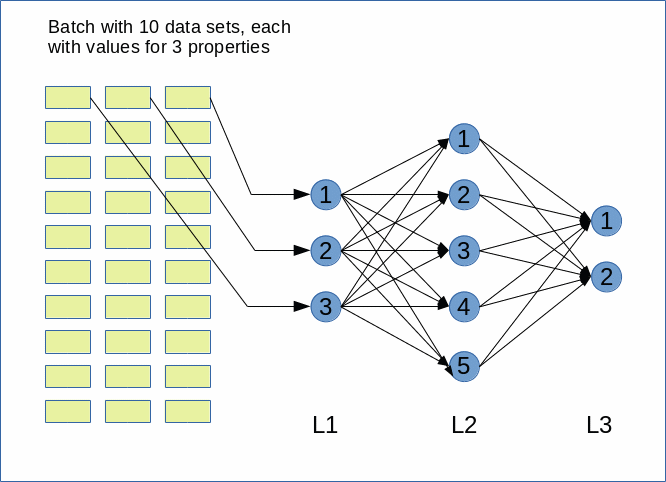Our batch has 10 data sets of test data for our ANN. Each data set describes 3 properties; thus the input layer "L1" has 3 nodes. Our "hidden" layer "L2" shall have 5 nodes and our output layer "L3" only 2 nodes.

# Weight matrices and propagation operations

Now, let us define some simple matrices with input vectors for transportation and weight-matrices for the layer-connections with Python. All activation functions shall in our simple example just perform a multiplication by 1.

The input to layer "L1" - i.e. a batch of 10 data sets - shall be given by a matrix of 10 lines (dimension 1) and 3 columns (dimension 2)

```import numpy as np
import scipy
import time

A1 = np.ones((10,3))
A1[:,1] *= 2
A1[:,2] *= 4
print("\nMatrix of input vectors to layer L1:\n")
print(A1)

print("\nMatrix of input vectors to layer 1:\n")
print(A1)

Matrix of input vectors to layer 1:

Matrix of input vectors to layer L1:

[[1. 2. 4.]
[1. 2. 4.]
[1. 2. 4.]
[1. 2. 4.]
[1. 2. 4.]
[1. 2. 4.]
[1. 2. 4.]
[1. 2. 4.]
[1. 2. 4.]
[1. 2. 4.]]
```

We now define the weight matrix "W1" for transport between layer L1 and layer L2 as:

```W1= np.random.randint(1, 10, 5*3)
W1 = w1.reshape(5,3)
print("\nMatrix of weights W1(L1, L2) :\n")
print(W1)

Matrix of weights W1(L1, L2) :

[[3 8 5]
[4 9 7]
[4 1 3]
[8 8 4]
[3 1 9]]

```

We set the first dimension to the number of nodes in the target layer (L2) and the second dimension to the number of nodes in the lower preceding layer (here: L1). Expressed in the shape notation for an array "(dimension 1, dimension 2, ...) ":

Shape of W : (dim 1, dim 2) = (node number of target layer, node number of preceding layer)

If we now tried a numpy "dot"-operation for our matrices

```A2 = np.dot(W1, A1)
```

we would get an error:

ValueError: shapes (5,3) and (10,3) not aligned: 3 (dim 1) != 10 (dim 0)

Well, this is actually consistent with the numpy documentation: The last dimension of "W1" should be consistent with the second to last (=first) dimension of "strong>A1".

What we need to do here is to transpose the matrix of our input vectors for our multiple data sets:

```A1 = np.transpose(A1)
print("\nMatrix of output vectors of layer 1 to layer 2:\n")
print(A1)

Matrix of output vectors of layer 1 to layer 2:

[[1. 1. 1. 1. 1. 1. 1. 1. 1. 1.]
[2. 2. 2. 2. 2. 2. 2. 2. 2. 2.]
[4. 4. 4. 4. 4. 4. 4. 4. 4. 4.]]

```

Then

```A2 = np.dot(W1, A1)
print("\n\nA2:\n")
print(A2)

A2:

[[39. 39. 39. 39. 39. 39. 39. 39. 39. 39.]
[50. 50. 50. 50. 50. 50. 50. 50. 50. 50.]
[18. 18. 18. 18. 18. 18. 18. 18. 18. 18.]
[40. 40. 40. 40. 40. 40. 40. 40. 40. 40.]
[41. 41. 41. 41. 41. 41. 41. 41. 41. 41.]]

```

Now, we need a weight matrix W2 between layer L1 and layer L2:

```W2= np.random.randint(1, 3, 2*5)
W2 = W2.reshape(2,5)
print("\n\nW2:\n")
print(W2)

W2:
[[2 2 1 2 2]
[2 2 2 2 1]]

```

We followed the same policy - and used the number of nodes in target layer L2 as the first dimension of the weight array.

Now, do we have to to anything with array A2, if we want to use it as an input for the next matrix operation ?

```A3 = np.dot(W2, A2)
```

No, we do not need to change anything! "A2" has the required form, already. The second to last dimension in "A2" is 5 in our example - as is the last dimension in "W2"!

So, the following will work directly :

```A3 = np.dot(W2, A2)
print("\n\nA3:\n")
print(A3)

A3:

[[358. 358. 358. 358. 358. 358. 358. 358. 358. 358.]
[335. 335. 335. 335. 335. 335. 335. 335. 335. 335.]]

```

You can try out our complete "propagation" code in a Jupyter notebook cell.

```import numpy as np
import scipy
import time

A1 = np.ones((10,3))
A1[:,1] *= 2
A1[:,2] *= 4
print("\nMatrix of input vectors to layer L1:\n")
print(A1)

W1= np.random.randint(1, 10, 5*3)
W1 = W1.reshape(5,3)
print("\nMatrix of weights W1(L1, L2) :\n")
print(W1)

A1 = np.transpose(A1)
print("\nMatrix of output vectors of layer L1 to layer L2:\n")
print(A1)

A2 = np.dot(W1, A1)
print("\n\nA2:\n")
print(A2)

W2= np.random.randint(1, 3, 2*5)
W2 = W2.reshape(2,5)
print("\n\nW2:\n")
print(W2)

A3 = np.dot(W2, A2)
print("\n\nA3:\n")
print(A3)
```

# Conclusion

The first thing we learned is that matrix operations on simple vectors can be extended to a set of vectors, i.e. a matrix. In the case of ANNs one dimension of such an (numpy) array would cover the number of data sets of a typical input mini-batch. The other dimension would cover the "properties" of the input data.

The second thing we saw is that the input matrix to an ANN often must be transposed to work together with weight matrices, if such matrices follow the policy that the first dimension is given by the number of cells in the target layer and the second dimension by the number of nodes of the preceding layer.

The weighted input to the first target layer is then given by a matrix "dot"-operation on the transposed input matrix. The outcome is a matrix where the first dimension is defined by the number of nodes of the target layer and the second dimension the number of data sets. This has already the correct form for a further propagation to the next layer. Note that the application of an activation function to each of the matrix elements would not change the required arrangement of matrix data!

After the input layer we, therefore, can just continue to directly apply weight matrices to output matrices by a numpy-"dot"-operation. The weight matrix structure just should reflect our shape policy of

(dim1, dim2) = (node number of target layer, node number of preceding layer)

.

In the next articles we shall use these insights to build a Python class for the setup and training of a simple ANN with one or two hidden layers for the analysis of the famous MNIST dataset.